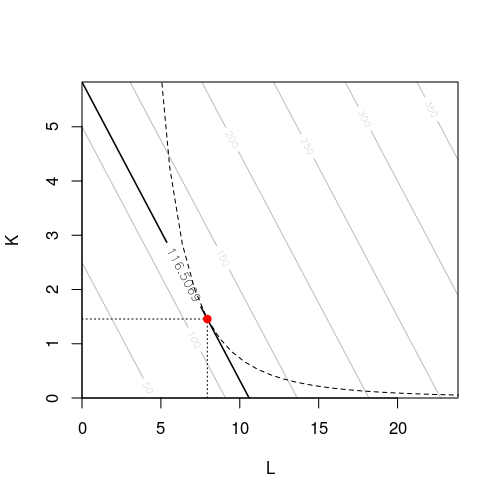## Exam 1

1. #### Question

A firm has the following production function: $F(K,L)= K L^{3}.$ The price for one unit of capital is $p_K = 20$ and the price for one unit of labor is $p_L = 11$. Minimize the costs of the firm considering its production function and given a target production output of 730 units.

How high are in this case the minimal costs?

#### Solution

Step 1: Formulating the minimization problem. \begin{aligned} \min_{K,L} C(K,L) & = p_K K + p_L L\\ & = 20 K + 11 L\\ \mbox{subject~to:} & F(K,L) = Q \\ & K L^{3} = 730 \end{aligned} Step 2: Lagrange function. \begin{aligned} \mathcal{L}(K, L, \lambda) & = C(K, L) - \lambda (F(K, L) - Q) \\ & = 20 K + 11 L - \lambda (K L^{3} -730) \end{aligned} Step 3: First order conditions. \begin{aligned} \frac{\partial {\mathcal {L}}}{\partial K} & = 20 - \lambda L^{3} = 0\\ \frac{\partial {\mathcal {L}}}{\partial L} & = 11 - {3} \lambda K L^{3 - 1} = 0 \\ \frac{\partial {\mathcal {L}}}{\partial \lambda} & = -(K L^{3}-730) = 0 \end{aligned} Step 4: Solve the system of equations for $K$, $L$, and $\lambda$.

Solving the first two equations for $\lambda$ and equating them gives: \begin{aligned} \frac{20}{L^{3}} & = \frac{11}{{3} K L^{3 - 1}}\\ K & = \frac{11}{3 \cdot 20} \cdot L^{3 - (3 - 1)}\\ K & = \frac{11}{60} \cdot L \end{aligned} Substituting this in the optimization constraint gives: \begin{aligned} K L^{3} & = 730\\ \left(\frac{11}{60}\cdot L \right) L^{3} & = 730\\ \frac{11}{60} L^{4} & = 730\\ L & = \left(\frac{60}{11} \cdot 730\right)^{\frac{1}{4}} = 7.9436547 \approx 7.94\\ K & = \frac{11}{60} \cdot L = 1.4563367 \approx 1.46 \end{aligned}

The minimal costs can be obtained by substituting the optimal factor combination in the objective function: \begin{aligned} C(K, L) & = 20 K + 11 L\\ & = 29.126734 + 87.380201 \\ & = 116.506935 \approx 116.51 \end{aligned}

Given the target output, the minimal costs are $116.51$.